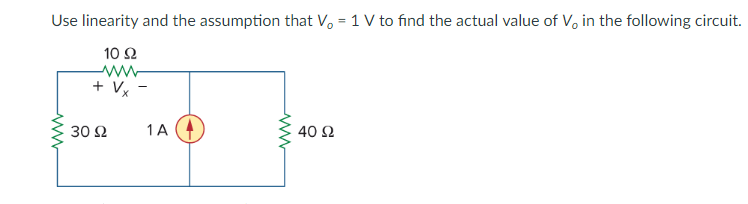# Use linearity and the assumption that Vo 1 V to find the actual value of Vo in the following circuit.10Ω30 Ω1A40Ω

Question

Use linearity and the assumption that Vo = 1 V to find the actual value of Vo in the following circuit. (circuit is displayed in attached picture)help_outlineImage TranscriptioncloseUse linearity and the assumption that Vo 1 V to find the actual value of Vo in the following circuit. 10Ω 30 Ω 1A 40Ω fullscreen
check_circle

Step 1

A linear circuit is one whose output is linearly related or in other words which is directly proportional to its input.

Let us consider the voltage Vx = 1 volt, then the current across 10Ω resistor will be:

Step 2

Thus, the voltage across 30Ω and 10Ω resistance will be:

V30-10Ω = 0.1 X 40= 4 volts.

Then the current through 40 Ω resistance is shown on the board:

And this would require a current source which is equal to -0.1-0.1 =0.2 amps.

Step 3

Since the given current source is 1 A which can be written as -5(-0.2) then the voltage Vx would be:

Vx = -5 x1 = -5 volts

Now if Vo= 1 V, then the current through the 2 Ω and 4 Ω resist...

### Want to see the full answer?

See Solution

#### Want to see this answer and more?

Solutions are written by subject experts who are available 24/7. Questions are typically answered within 1 hour.*

See Solution
*Response times may vary by subject and question.
Tagged in

### Electrical Engineering